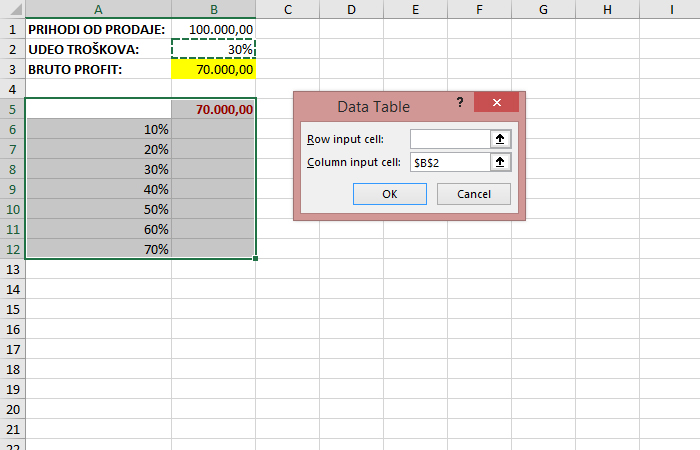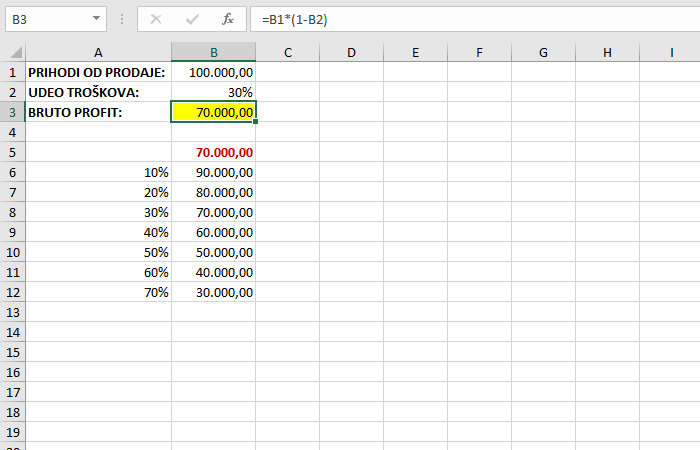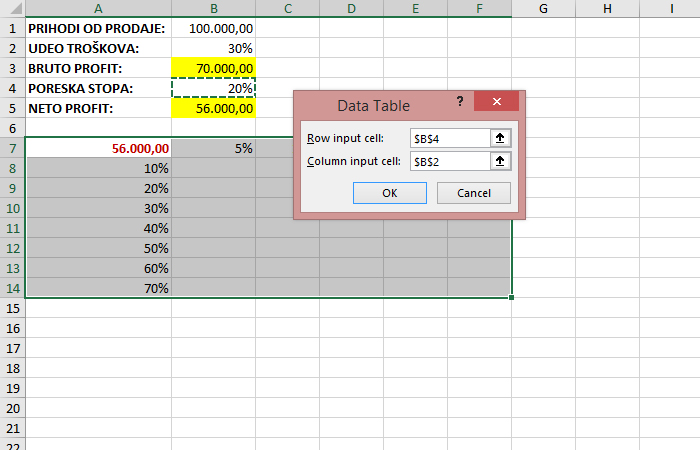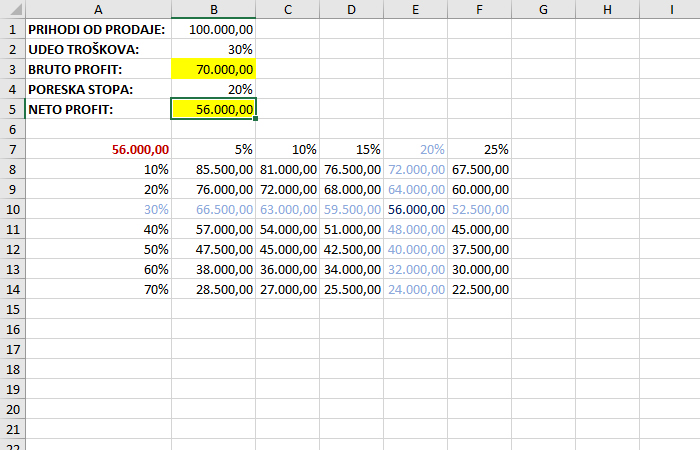# Data Table

The Data Table is an old and somewhat neglected Excel option that allows you to see the results of applying different scenarios within a table. For a given formula and criteria, it returns alternative values ​​of that formula. Criteria can be drawn from rows or columns, and there is the possibility of a report containing two groups of criteria. This is perhaps a better tool than a scenario if we perform simpler analyzes …

We start from a simple table that contains sales revenue (PRIHODI OD PRODAJE), cost share (UDEO TROŠKOVA) and gross profit (BRUTO PROFIT: revenue * (1-rate cost share)). If we want to see what will happen to gross profit due to the change in the rate of share of costs, we will create below the table where in the rows of the first column are different rates, and in the header of the second column a reference to the formula for calculating gross profit. Select the table (A5: B12) and find the What-If Analysis menu in the Data ribbon. The Data Table option should be selected in this menu. In the Column Input Cell field, you should enter a reference to the cell in which the cost share rate is located. That way Excel will know which values ​​in the formula to change for different scenarios. If the data were in columns (transposed table), we would enter the value in the Row Input Cell field.After entering criterium and clicking OK we’ll confirm the action that will fill the table.Let’s create a slightly more complex scenario. Let’s add in the original table the tax rate (PORESKA STOPA), as well as the value of net profit (NETO PROFIT) that is obtained when taxes are deducted from gross profit (this is valid in practice when we finance business from our own sources). Then the analysis table will look a little different. We state the values ​​of one rate in rows, and the values ​​of the other in columns. At the intersection of rows and columns there is a reference to the formula used to calculate net profit. Select the table (A7: F14) and restart the Data Table option. Now the value of the first rate should be entered in Column Input Cell, and the value of the second in Row Input Cell.After confirming the action, we will get a table with the values ​​of the application of the two criteria.